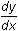Module 13 - Implicit Differentiation Introduction | Lesson 1 | Lesson 2 | Self-Test Self Test Consider the implicit relation x2y + xy2 = 6. Graph the relation. Find. Find the slope of the tangent at the point (1,2). Find the point where the tangent line is horizontal. Find the point where the tangent line is vertical. Click here to check your answers. < Back ©Copyright 2007 All rights reserved. | Trademarks | Privacy Policy | Link Policy